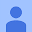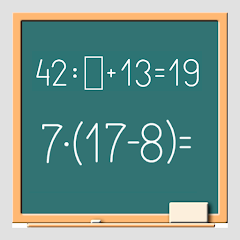# Math on chalkboard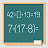2.4
1.46K reviews
100K+Everyone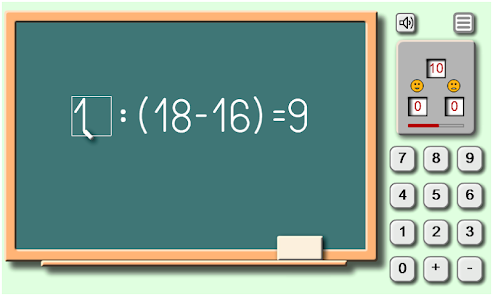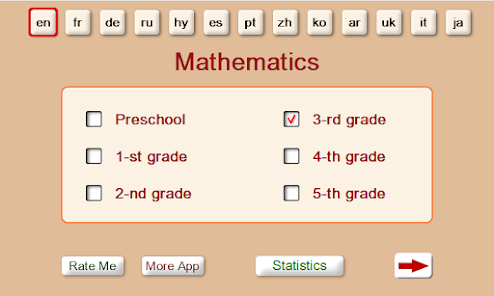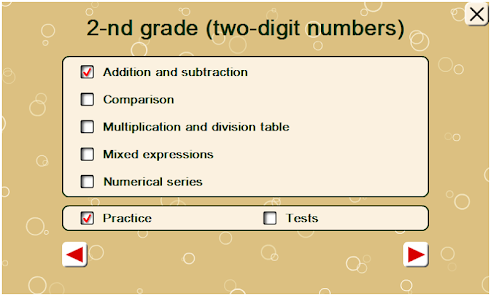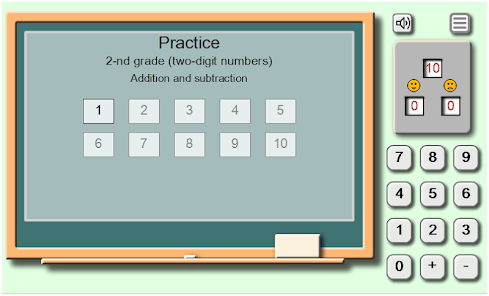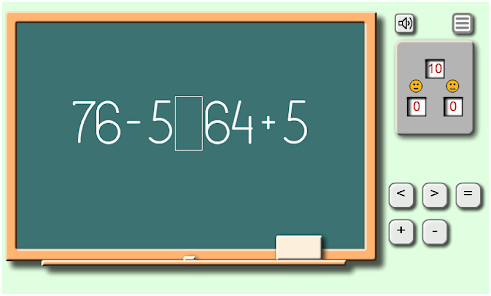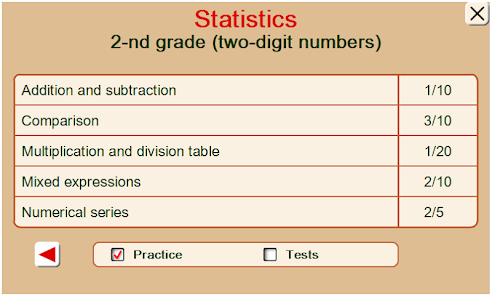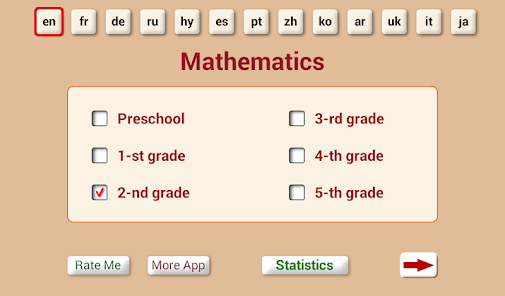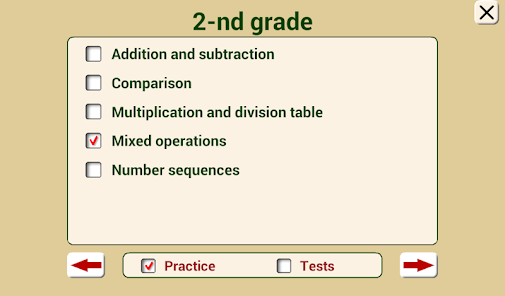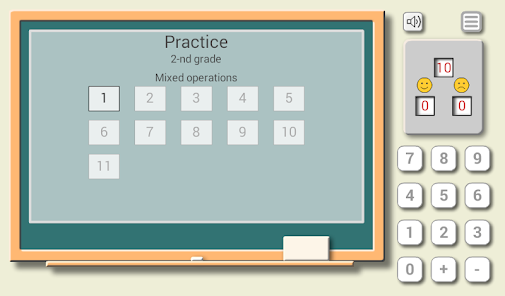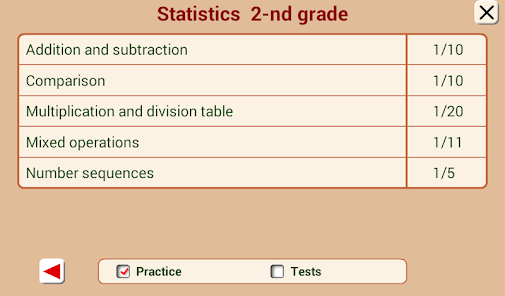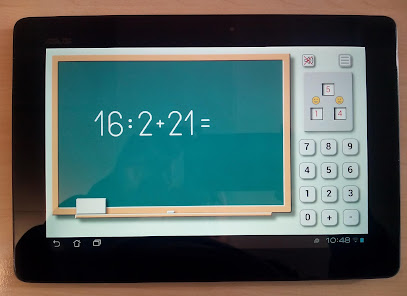Math application "Math on a chalkboard" is presented as an animated game that helps you to enhance mental arithmetic skills, facilitates the memorization of multiplication and division tables. Good animation gives you a feeling of being in the classroom, in front of the board.
The application consists of 6 age categories with relevant topics:

Preschool (numbers up to 10)
• Comparison
• Number sequences

1st grade (numbers up to 20)
• Comparison
• Number sequences

• Comparison
• Multiplication and division table
• Mixed operations
• Number sequences

• Comparison
• Mixed operations
• Operations with fractions
• Compare fractions
• Number sequences

• Mixed operations
• Comparison
• Operations with fractions
• Compare fractions
• Number sequences

• Mixed operations
• Comparison
• Operations with fractions
• Compare fractions
• Operations with decimals
• Compare decimal numbers
• Number sequences

"Addition and subtraction" are mathematical calculations with bit transition or without transition.
"Comparison" includes a comparison of the numbers, as well as results of mathematical calculations.
"Multiplication and division table" will allow children to learn the multiplication table, adults may also refresh their knowledge of math.
"Mixed expressions" provides mathematical exercises with the use of plus, minus, multiplication, and division, as well as expressions with brackets and examples in few actions.
"Number sequences" is a number series where you must find the pattern and insert the missing number.

All the math exercises are grouped by levels. The higher is the level, the more difficult the is calculation. To move to the next level you must correctly solve all the exercises of the current level.
After selecting the age category and topic, you can move on to practical exercises ("Practice") or test tasks ("Tests").

The section "Practice" contains many different kinds of mathematical exercises that help to develop the skills of oral accounts. It is job training which helps you to improve your knowledge of math, and then easily pass the tests of this application.
In the section "Tests" for each exercise, 15 seconds are given. If the result is not entered within 15 seconds, the answer is considered incorrect. This highly improves the ability to quickly finding solutions to mathematical expressions.

The scoreboard indicates the total number of exercises on the current level and the number of correct and incorrect answers.
The application is made in the form of a simulator. Regular, repeated exercise will give good results in mastering arithmetic calculations such as addition, subtraction, multiplication, division, and will help quickly learn the multiplication and division table. Because many arithmetic and mathematical tasks require excellent knowledge of multiplication and division tables.

Features of the application:
• 6 age categories;
• more than 4,000 math exercises on different topics;
• multi-level system with increasing difficulty;
• animated chalkboard for self-training;
• sound effects;
• multi-language support;
• statistics showing the number of levels on the topics.

Supported languages:
English, Armenian, Spanish, Italian, Chinese, German, Portuguese, Russian, Ukrainian, French, Korean, Japanese, Arabic.
Updated on
Dec 31, 2018

## Data safety

Safety starts with understanding how developers collect and share your data. Data privacy and security practices may vary based on your use, region, and age. The developer provided this information and may update it over time.No data shared with third partiesNo data collected
2.4
1.46K reviews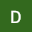Dron Giri
February 24, 2021
Osm and very nice app to improve maths so if you are in this app download now
13 people found this review helpful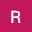Rabiya Mb
September 16, 2020
Waste game 😡😡😡😡 please don't download this game friends, I have gave one star ⭐because I like chalkboard writing sound
4 people found this review helpful